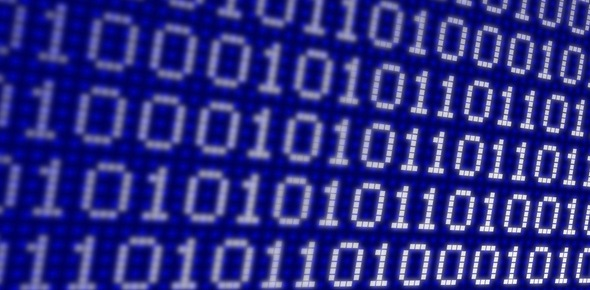# Binary Code First Short Test

Approved & Edited by ProProfs Editorial Team
The editorial team at ProProfs Quizzes consists of a select group of subject experts, trivia writers, and quiz masters who have authored over 10,000 quizzes taken by more than 100 million users. This team includes our in-house seasoned quiz moderators and subject matter experts. Our editorial experts, spread across the world, are rigorously trained using our comprehensive guidelines to ensure that you receive the highest quality quizzes.
| By Oflodac
O
Oflodac
Community Contributor
Quizzes Created: 13 | Total Attempts: 54,648
Questions: 5 | Attempts: 2,981SettingsBinary code is a coding system using the binary digits 0 and 1 to represent a letter, digit, or other character in a computer or other electronic device. The Binary Code First Short Test below is designed to help you get a better understanding of your course work before the exam. Give it a shot!

• 1.

### How many bits are in a byte?

• A.

1

• B.

8

• C.

10

• D.

None

B. 8
Explanation
A byte is a unit of digital information that consists of 8 bits. Each bit can represent either a 0 or a 1, allowing for a total of 256 possible combinations (2^8). Therefore, the correct answer is 8.

Rate this question:

• 2.

### 01001101 represents the letter M in

• A.

Decimal.

• B.

• C.

Kilobytes.

• D.

Binary.

D. Binary.
Explanation
The given binary code "01001101" represents the letter M in binary. Binary is a number system that uses only two digits, 0 and 1, to represent all numbers and characters. In this case, the binary code "01001101" corresponds to the letter M in the binary system.

Rate this question:

• 3.

### Which of the following is in correct order, from smallest to largest?

• A.

Byte, Kilobyte, Megabyte, Gigabyte, Terabyte

• B.

Megabyte, Kilobyte, Terabyte, Gigabyte, Byte

• C.

Byte, Kilobyte, Terabyte, Megabyte, Gigabyte

• D.

Terabyte, Gigabyte, Megabyte, Kilobyte, Byte

A. Byte, Kilobyte, Megabyte, Gigabyte, Terabyte
Explanation
The correct order, from smallest to largest, is Byte, Kilobyte, Megabyte, Gigabyte, Terabyte. This is because each unit is a multiple of 1024 of the previous unit. A Kilobyte is 1024 Bytes, a Megabyte is 1024 Kilobytes, a Gigabyte is 1024 Megabytes, and a Terabyte is 1024 Gigabytes. Therefore, Byte is the smallest unit and Terabyte is the largest unit.

Rate this question:

• 4.

### In binary code, an 8-bit unit is called a ____________________.

Byte
byte
Explanation
In binary code, an 8-bit unit is called a byte. The term "byte" is used to refer to a sequence of 8 bits, which is the basic unit of storage in computer systems. It is commonly used to represent characters, numbers, and other data in computer programming. The lowercase "byte" is also accepted as a correct term for an 8-bit unit in binary code.

Rate this question:

• 5.

### 2,000,000 bytes=________________MB

2
2mb
2 mb
2MB
2 MB
Explanation
The given answer is correct because 2,000,000 bytes is equal to 2 megabytes (2MB). The "MB" stands for megabytes, which is a unit of digital storage.

Rate this question:Back to top